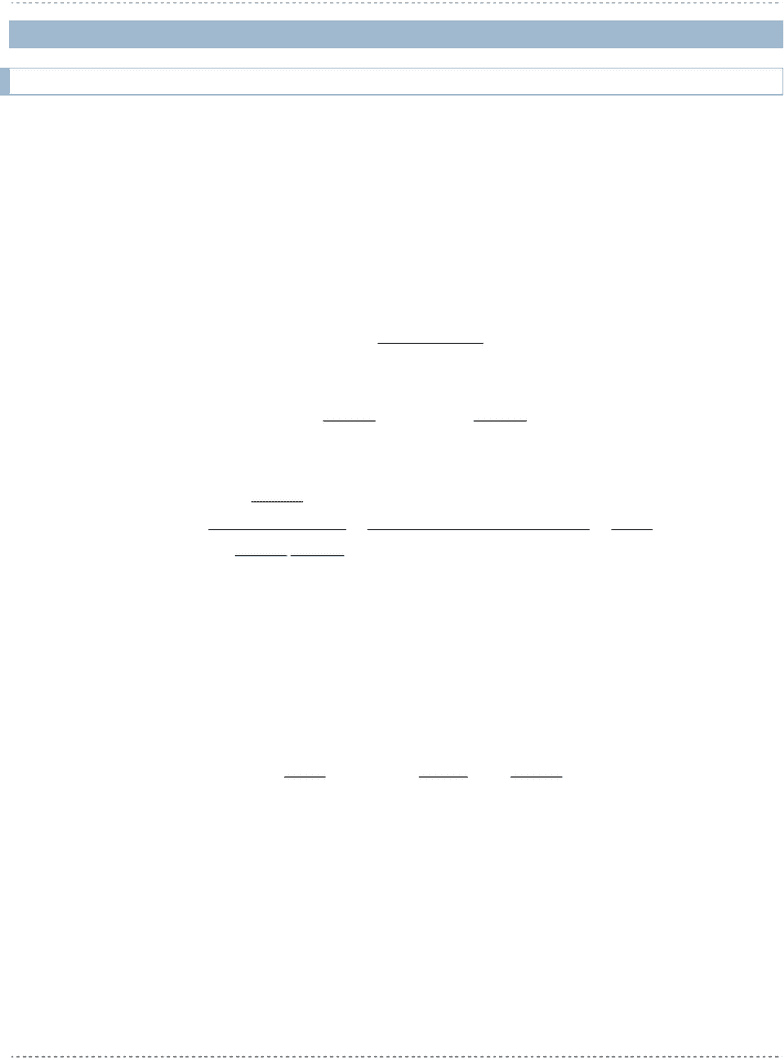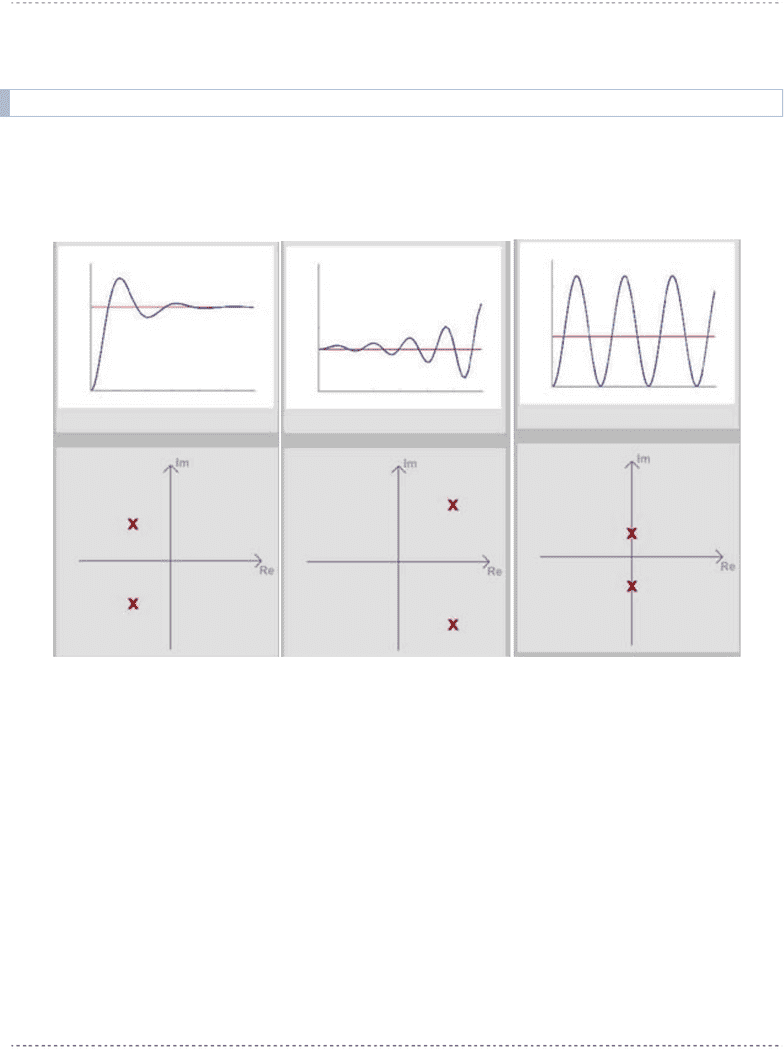# ELE 639 Lecture Notes - Lecture 2: Edward Routh, Adolf Hurwitz, Imaginary Number

77 views25 pagesELE/BME639 Course Notes Winter 2011
Page 33
2 Stability
2.1 General Definition of Stability
Stability is an implicitly stated control objective. Intuitively, a closed loop system is stable if it does not
"blow up". For introduction, see Online Tutorials - sections on Basic Concepts and on Stability.
Recall from ELE532 that mathematically, stability is related to the location of the closed loop system
transfer function poles.
Definition: A system is stable in BIBO sense if, for every bounded input, the output remains bounded.
Consider now the transfer function of a basic closed loop system:
)()(1
)(
)( sHsG
sG
sGcl
)(
)(
)( sD
sN
sG
G
G
)(
)(
)( sD
sN
sH
H
H
)(
)(
)()()()(
)()(
)(
)(
)(
)(
1
)(
)(
)( sQ
sN
sNsNsDsD
sDsN
sD
sN
sD
sN
sD
sN
sG
HGHG
HG
H
H
G
G
G
G
cl
Equation 2-1
Characteristic equation of the closed loop system is:
0)(
sQ
Equation 2-2

tp
n
iinatural
forcednatural
forcednatural
jj
j
n
ii
i
cl
i
eKty
tytysYLty
sYsYsY
ps
K
ps
K
sU
sQ
sN
sY
sUsGsY
1
1
1
)(
)()()()(
)()()(
)(
)(
)(
)(
)()()(
Equation 2-3
Definition: Suppose that the closed loop system has a transfer function . The system is stable if, and
only if, the poles of  shown in Equation 2-3 have strictly negative real parts: 0.
Unlock document

This preview shows pages 1-3 of the document.
Unlock all 25 pages and 3 million more documents.ELE/BME639 Course Notes Winter 2011
Page 34
To determine this condition analytically (as opposed to a numerical solution, such as provided by
MATLAB) a Criterion of Stability is defined - the Routh Test for Stability.
2.2 Locations in s-Plane vs. Time Response
Figure 2-1below shows three possible s-Plane locations for a pair of complex conjugate poles. Recall that
the pair of complex conjugate poles results in oscillatory time response, where the Real part of the pole
determines the decay rate of the response, while the Imaginary part of the pole determines the frequency
of oscillations.
Figure 2-1 Pole Locations vs. Time response
The first panel of Figure 2-1illustrates a stable response, the second panel illustrates an unstable response,
and the third panel illustrates a marginally stable response. The system respective behaviours will be
labeled as Stable, Unstable and Marginally Stable. Because we have the pair of complex conjugate poles,
the second, Ustable, case results in an oscillatory instability.
Note that these three behaviours can also be applied to a case where the system pole(s) are real. Real
poles result in transient(s) of exponential form. When 0, we will have a combination of
exponential decays, when 0 we will have a constant (step) response, and when 0, we will
have an exponentially increasing (but of single polarity, not oscillating) unstable response. Thus this case
is referred to as monotonic instability.
Refer to Online Tutorials for more on System Stability:
http://echo.ryerson.ca/flashcom/applications/controlsys/
Unlock document

This preview shows pages 1-3 of the document.
Unlock all 25 pages and 3 million more documents.ELE/BME639 Course Notes Winter 2011
Page 35
2.3 Stability in s-Domain: The Routh-Hurwitz Criterion of Stability
The original Criterion was formulated in a paper published in 1877 by Edward Routh, English
mathematician born in Upper Canada (now Quebec). In 1895 German mathematician Adolf Hurwitz
formulated the Criterion in its today's form, based on theory of polynomials. This is why the Criterion
bears both their names.
2.3.1 Necessary Condition for Stability
Definition: The Necessary Condition for Stability requires that all coefficients of the Characteristic
Equation polynomial are present and have the same sign. (In practice, it means that they should all be
positive, as negative signs would correspond to a negative Controller gain. A control system with a
negative gain is not practical as it would do exactly the opposite to the Command (Reference) input).
Where does the Necessary Condition come from? Consider the following. Once the Characteristic
Equation is factorized into a ZPK form, it will consist of two types of factors, 1st and 2nd order, as shown.
If the roots of these factors are in the LHP (i.e. the Stable Region of the s-plane), then the resulting
coefficients in these factors will be positive:
)(
)(
2kk
j
bsas
as
- stable factors
For example, 5
and 314
are factors corresponding to stable pole locations (-5 and -
1.5+j3.43, -1.5-j3.43, respectively), while 5
, 314
are factors corresponding to unstable pole
locations (+5 and +1.5+j3.43, +1.5-j3.43 respectively). Also note that one of the two poles corresponding
to the 314
factor is unstable (poles are: -5.53,+2.53).
0)(
)()()(
01
2
2
1
1
2
asasasasasQ
bsasassQ
n
n
n
n
kkk
jj
Equation 2-4
Conclusion 1: if only stable factors are present in Equation 2-4, after multiplication, the polynomial form of
the characteristic equation will have all powers of s terms present and all coefficients will be positive.
There is no possibility of having a negative sign or of a term cancellation resulting in a missing power of s,
since all factor signs were positive.
Conclusion 2: Any negative signs or any terms that are missing indicate presence of a factor or factors
describing unstable pole location(s).
Example:
013)( 3sssQ roots are: 0.16 + j1.75, 0.16 - j1.75, -0.3222 (conjugate pair unstable)
Unlock document

This preview shows pages 1-3 of the document.
Unlock all 25 pages and 3 million more documents.

## Get access

\$8 USD/m\$10 USD/m
Billed \$96 USD annuallyHomework Help
Study Guides
Textbook Solutions
Class Notes
Textbook Notes
Booster Class